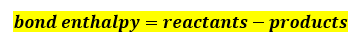# Problem: The table below shows the average bond energies for some common bonds among carbon, hydrogen, and oxygen.BondBond energy(kJ/mol)C - C347C - H414C - O360C = O799H - H436H - O464O - O142O = O498Calculate ΔHrxn for the combustion of octane by using enthalpies of formation from the transition above.

###### FREE Expert Solution

To calculate ΔHrxn using bond energies, we will use the following equation:Write the combustion reaction of octane:

• Hexane is a a hydrocarbon composed only of carbon and hydrogen.
• The prefix oct- means 8 carbons.
• General Formula: CnH2n+2    where n = number of carbons
• Hexane: C8H18

Balanced Combustion reaction:###### Problem Details

The table below shows the average bond energies for some common bonds among carbon, hydrogen, and oxygen.

 Bond Bond energy(kJ/mol) C - C 347 C - H 414 C - O 360 C = O 799 H - H 436 H - O 464 O - O 142 O = O 498

Calculate ΔHrxn for the combustion of octane by using enthalpies of formation from the transition above.